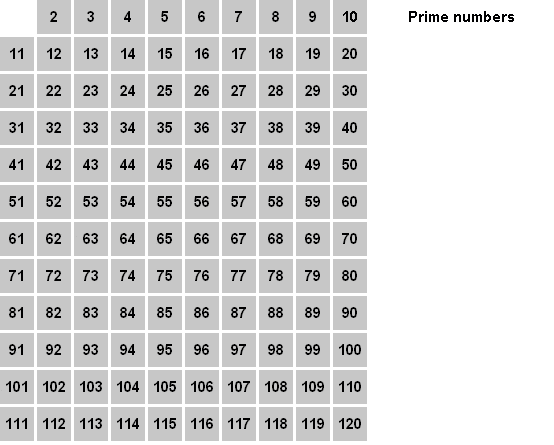# 2. Sieve of Eratosthenes

Print all the prime numbers between 1 and 1024.

Method to find all the prime numbers less than or equal to a given integer n:

1. Create a list of consecutive integers from two to n: (2, 3, 4, ..., n).
2. Initially, let p equal 2, the first prime number.
3. Strike from the list all multiples of p less than or equal to n. (2p, 3p, 4p, etc.).
4. Find the first number remaining on the list after p (this number is the next prime); replace p with this number.
5. Repeat steps 3 and 4 until p2 is greater than n.
6. All the remaining numbers in the list are prime.Now implement the algorithm using bit-twiddling instead of arrays. Do not use an array to store the "primeness" of a number. Use bits instead to store that infomation.